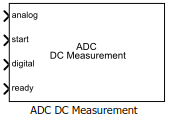Measure DC performance metrics of ADC output

Since R2019a

•Libraries:
Mixed-Signal Blockset / ADC / Measurements & Testbenches

## Description

The ADC DC Measurement block measures ADC DC performance metrics such as offset error, gain error, integral nonlinearity (INL), and differential nonlinearity (DNL). You can use ADC DC Measurement block to validate the ADC architectural models provided in Mixed-Signal Blockset™, or you can use an ADC of your own implementation.

## Ports

### Input

expand all

Data Types: `double`

External conversion start clock, specified as a scalar. The analog to digital conversion process starts at the rising edge of the signal at the start port.

Data Types: `double`

Converted digital signal from an ADC, specified as a scalar.

Data Types: `fixed point` | `single` | `double` | `int8` | `int16` | `int32` | `uint8` | `uint16` | `uint32`

Indicates whether the analog to digital conversion is complete, specified as a scalar.

Data Types: `double`

## Parameters

expand all

Number of physical bits in ADC, specified as a unitless positive real integer. Number of bits must match the resolution specified in the ADC block.

#### Programmatic Use

• Use `get_param(gcb,'NBits')` to view the current Number of bits.

• Use `set_param(gcb,'NBits',value)` to set Number of bits to a specific value.

Frequency of the start conversion clock of the ADC, specified as a positive real scalar in hertz. Start conversion frequency must match the frequency of the start conversion clock of the ADC block. This parameter is used to calculate Recommended simulation stop time.

#### Programmatic Use

• Use `get_param(gcb,'Frequency')` to view the current value of Start conversion frequency.

• Use `set_param(gcb,'Frequency',value)` to set Start conversion frequency to a specific value.

Dynamic range of the ADC, specified as a 2-element vector in V. The two vector elements represent the minimum and maximum values of the dynamic range, from left to right.

#### Programmatic Use

• Use `get_param(gcb,'InputRange')` to view the current value of Input range.

• Use `set_param(gcb,'InputRange',value)` to set Input range to a specific value.

Delays measurement analysis to avoid corruption by transients, specified as a nonnegative real scalar in seconds.

#### Programmatic Use

• Use `get_param(gcb,'HoldOffTime')` to view the current value of Hold off time.

• Use `set_param(gcb,'HoldOffTime',value)` to set Hold off time to a specific value.

Minimum time the simulation must run to obtain meaningful results, specified as a positive real scalar in seconds.

For DC measurement, the simulation must run so that ADC can sample each digital code 10 times with the default error tolerance of `0.1`, assuming a ramp input that traverses the full scale range of the ADC over the period of simulation. Based on this assumption, the analog input frequency (fanalog), generated by the ADC Testbench block for the sawtooth waveform is set as:

`${f}_{\text{analog}}=\frac{StartFreq·ErrorTolerance}{{2}^{\left(Nbits+1\right)}}$`

where StartFreq is the frequency of the conversion start clock and Nbits is the resolution of the ADC.

So, the Recommended min. simulation stop time (s) (T) is calculated by using the formula: $T=\frac{1}{{f}_{\text{analog}}}+HoldOffTime$.

Data Types: `double`

Click to automatically set the Recommended min. simulation stop time (s) as the stop time of the Simulink® model.

Measure the differential nonlinearity (DNL) error and integral nonlinearity (INL) error using the endpoint method. This method uses the end points of the actual transfer function to measure the DNL and INL error.

Measure the differential nonlinearity (DNL) error and integral nonlinearity (INL) error using the best fit method. This method uses a standard curve fitting technique to find the best fit to measure the DNL and INL error.

Store detailed test results to a `struct` in the base workspace for further processing. By default, this option is not selected.

Name of the variable that stores detailed test results, specified as a character string.

#### Dependencies

This parameter is only available when Output result to base workspace is selected

#### Programmatic Use

• Use `get_param(gcb,'VariableName')` to view the current value of Workspace variable name.

• Use `set_param(gcb,'VariableName',value)` to set Workspace variable name to a specific value.

Click to plot measurement result for further analysis.